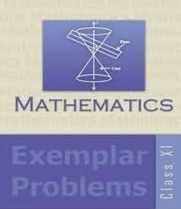# NCERT EXEMPLAR BOOK CLASS 11 Maths

## CBSE NCERT EXEMPLAR BOOK CLASS 11 Maths

NCERT Exemplar Books play a very important part for students for class 11 and 12. The concepts included in the books are very important since these concepts play a vital role for upcoming entrance examinations. It has been seen that questions from NCERT Exemplar Books are often asked in upcoming entrance examinations like JEE Main, JEE Advanced, NEET, AIIMS, etc.

## Why NCERT Exemplar Book Class 11 Maths?

NCERT Exemplar Book Class 11 Maths aims to provide a variety of quality problems with different difficulty levels. It contains Multiple Choice Question, Short Questions, Long Answer Questions, etc. In other words, it gives more importance to help the students understand the concept related to that particular chapter through logical reasoning, and not just memorise the formulae and write the exam.

The NCERT Exemplar Book Class 11 Maths contains 16 chapters. Each chapter starts with a brief description of the topic, which is followed by solved exercises and unsolved exercises. The unsolved exercises help the student to get a deeper understanding of the concept.

The questions and solutions given in NCERT Exemplar Book Class 11 Maths aim to create a solid base of the students. This will enable the students to solve critical problems.

Answers are written in the proper format, with each step in details, along with tables, graphs, and derivation wherever required. As a result, the students have a clear logic about the topic.

All the questions of NCERT Books are very important for higher secondary students and other competitive entrance exams. It has been analyzed that questions from NCERT Exemplar Book Class 11 Maths are frequently asked in entrance examinations like NEET, JEE Main, JEE Advanced, WBJEE, etc. Therefore, students must practice from NCERT Exemplar Book Class 11 Maths.

Labelled figures with proper illustrations, equations, tables, in-text formulae, derivations, etc., have been provided, wherever required, for effective understanding of the concept(s). As a result, the NCERT Exemplar Book Class 11 Maths helps the students to improve their mental ability and solve problems in a scientific manner.

View NCERT Exemplar Class 11 Physics

View NCERT Exemplar Class 11 Chemistry

View NCERT Exemplar Class 11 Biology